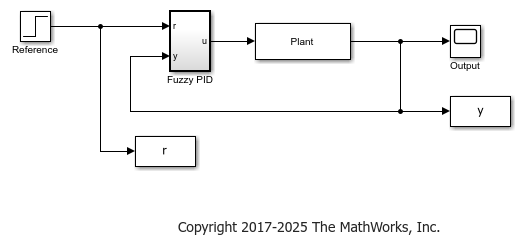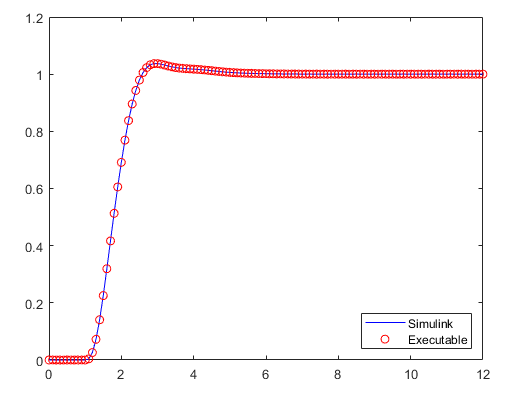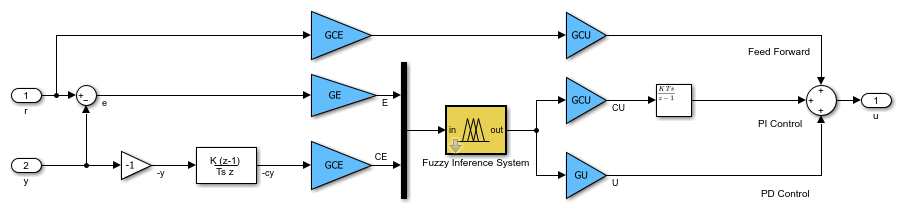Documentation

This is machine translation

Mouseover text to see original. Click the button below to return to the English version of the page.

Generate Code for Fuzzy System Using Simulink Coder

Generate Code for Fuzzy Inference System

By default, the Fuzzy Logic Controller block uses double-precision data for simulation and code generation. The `fuzzyPID` model is configured to use double-precision data. For more information on configuring your fuzzy inference system for code generation, see Fuzzy Logic Controller.

```mdl = 'fuzzyPID'; open_system(mdl) ```It is good practice to validate the performance of the system in Simulink. Run the simulation. The model saves the output response, `u`, to the MATLAB® workspace.

```sim(mdl) ```

To generate code for the model, use the `rtwbuild` function. For this example, suppress the Command Window output for the build process.

```set_param(mdl,'RTWVerbose','off') rtwbuild(mdl) ```
```### Starting build procedure for model: fuzzyPID ### Successful completion of build procedure for model: fuzzyPID ```

By default, Simulink Coder generates C code for a generic real-time target. To select a different target file and language, in the Configuration Parameters dialog box, modify the System target file and Language parameters, respectively.

The generated code is stored in a new `fuzzyPID_grt_rtw` folder in your current working folder. The name of this folder depends on the selected target file.

On a Windows® system, by default, an executable file named `fuzzyPID.exe` is also added to the current working folder. To generate code without compilation, in the Configuration parameters dialog box, select the Generate code only parameter before generating code.

Run the executable.

```if ispc status = system(mdl); else disp('The example only runs the executable on Windows system.'); end ```
```The example only runs the executable on Windows system. ```

After the executable completes successfully (`status = 0`), the software creates a `fuzzyPID.mat` data file that contains the simulation results.

You can compare the output response from the generated code, `rtw_y`, with the output from the Simulink simulation, `y`, using the following code:

```load fuzzyPID.mat plot(tout,y,'b-',rt_tout,rt_y,'ro') legend('Simulink','Executable','Location','Southeast') ```The result from the generated code matches the Simulink simulation.

You can also generate code for just the controller subsystem in this model. To do so, specify the subsystem when calling the `rtwbuild` function.

```rtwbuild([mdl '/Fuzzy PID']) ```
```### Starting build procedure for model: Fuzzy0 ### Successful completion of build procedure for model: Fuzzy0 ```

You can deploy generated code according to your application needs. For example, you can configure the properties of executable files and create static or dynamic libraries. For more information, see Build Process Workflow for Real-Time Systems (Simulink Coder).

Generate Code for Other Data Types

The Fuzzy Logic Controller block also supports single-precision and fixed-point data for simulation and code generation. In both cases, your resulting fuzzy system has decreased accuracy compared to an equivalent double-precision fuzzy system. Use:

• Single-precision data to reduce the memory footprint of your system.

• Fixed-point data if your target platform only supports fixed-point arithmetic.

To use one of these data types, set the Data type property of the block, and configure the other components in the model to use the same data type.

The `fuzzyPID_single` model is configured for single-precision data. Open the model.

```mdl2 = 'fuzzyPID_single'; open_system(mdl2) ```In this model, the Data type parameter of the Fuzzy Logic Controller block is set to `single`. The Fuzzy Logic Controller block automatically converts input signals to the specified data type. Also, the Simulate using parameter is set to `Code Generation`. The Simulate using option does not affect the code generation process. Instead, setting this option simulates your fuzzy system using the same code path used by generated code.

Generate code for this model.

```set_param(mdl2,'RTWVerbose','off') rtwbuild(mdl2) ```
```### Starting build procedure for model: fuzzyPID_single ### Successful completion of build procedure for model: fuzzyPID_single ```

Setting the Data type parameter of a Fuzzy Logic Controller block ensures that all the inference steps use the specified data type. However, depending on the configuration of other blocks in the model, some of the generated code can still use double-precision data.